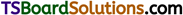# TS Inter 1st Year Chemistry Notes Chapter 6 Thermodynamics

Here students can locate TS Inter 1st Year Chemistry Notes 6th Lesson Thermodynamics to prepare for their exam.

## TS Inter 1st Year Chemistry Notes 6th Lesson Thermodynamics

→ In the study of thermodynamics, we divide the entire universe into two segments. One small segment of it is the one on which we conduct out studies. This is called system. The rest of the universe is considered ar-bitrarily as surroundings.

→ Systems are classified as open, closed and isolated systems.

→ Properties of the system are classified as intensive (non-depending on amount of matter), extensive (depending on amount of matter) and thermodynamic properties.

→ Thermodynamic properties are internal energy (E), enthalpy (H), Gibbs energy (G), entropy (S), work (W) etc.

→ Zeroth law deals with thermal equilibrium between the bodies. First law is concerned with conservation of energy, second law deals with spontaneity of a process and the third law deals with heat changes at absolute zero.

→ Application of first law of thermodynamics to chemical changes is known as thermo chemistry.→ We define the heat changes quantitatively for different processes. These are forma-tion, dissociation, combustion, netrtralizatipn, sublimation, ionization and dilution processes.

→ Heat changes can be experimentally deter-mined using calorimeters.

→ ΔG = ΔH – T ΔS. ΔG is negative for spontaneous and is positive for non-spontaneous and zero for equilibrium reactions.

→ Entropy (S), a thermodynamic property is introduced to express the molecular disorder of a chemical system.